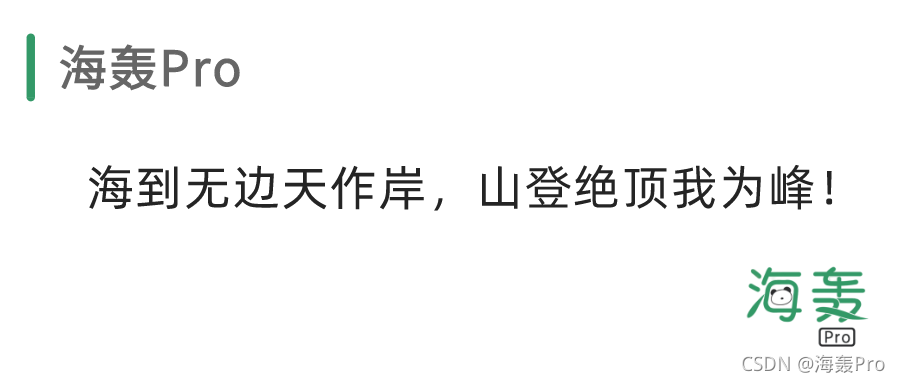# 【Dive into Deep Learning / 動手學深度學習】第二章 - 第一節：資料操作Hello！

# 2.1 資料操作

## 2.1.1. 入門

### torch.arange()

```python x = torch.arange(12) x```

tensor([ 0, 1, 2, 3, 4, 5, 6, 7, 8, 9, 10, 11])

### shape

```python x.shape```

torch.Size()

### numel()

```python x.numel()```

12

### reshape()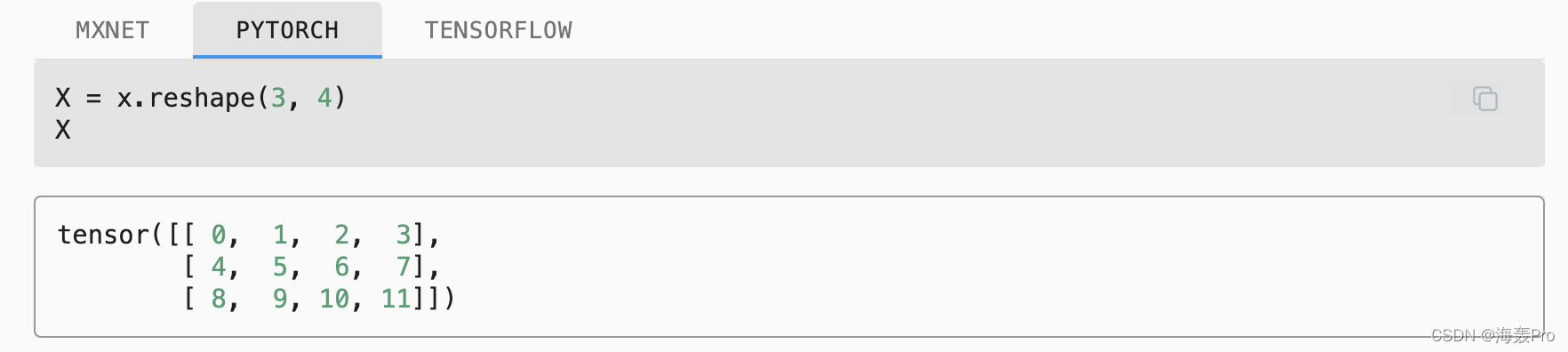### ones()

```python torch.ones((2, 3, 4))```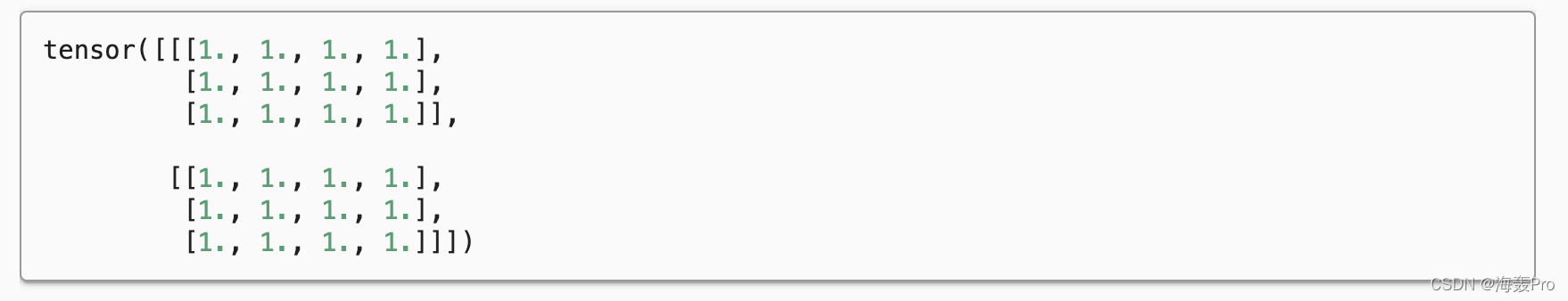### randn()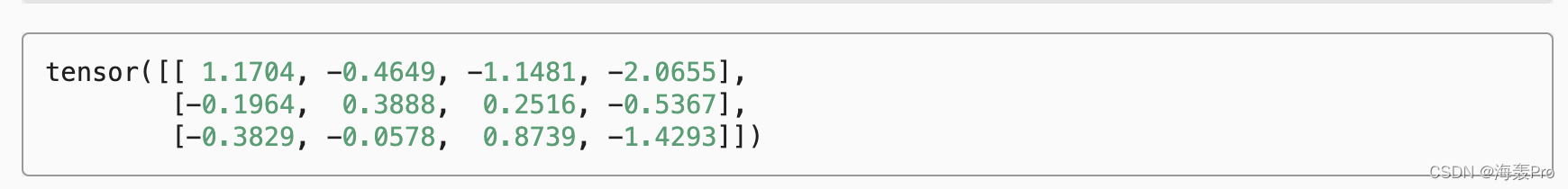## 2.1.2. 運算子

### +、-、、/、* 、exp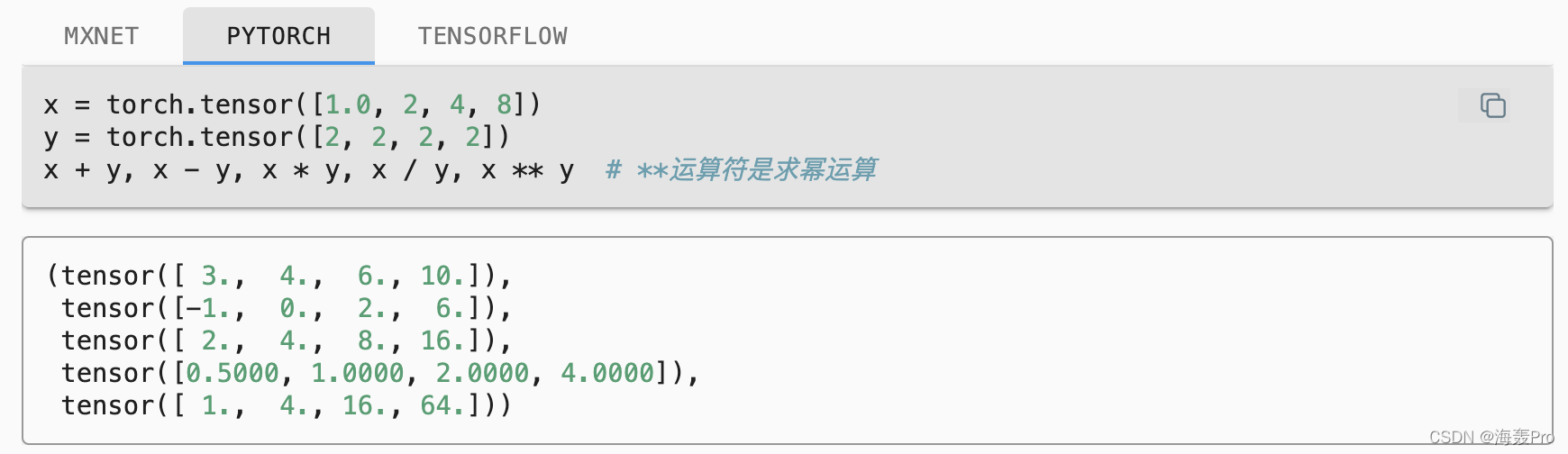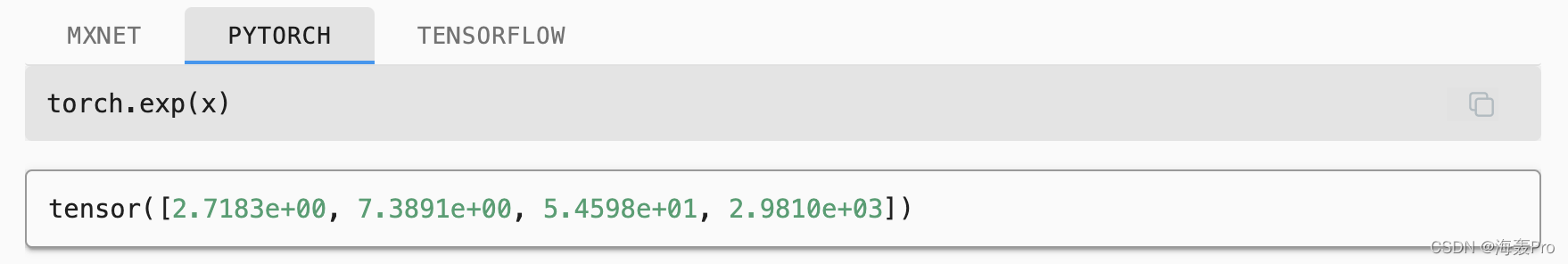### 多個張量連結（concatenate）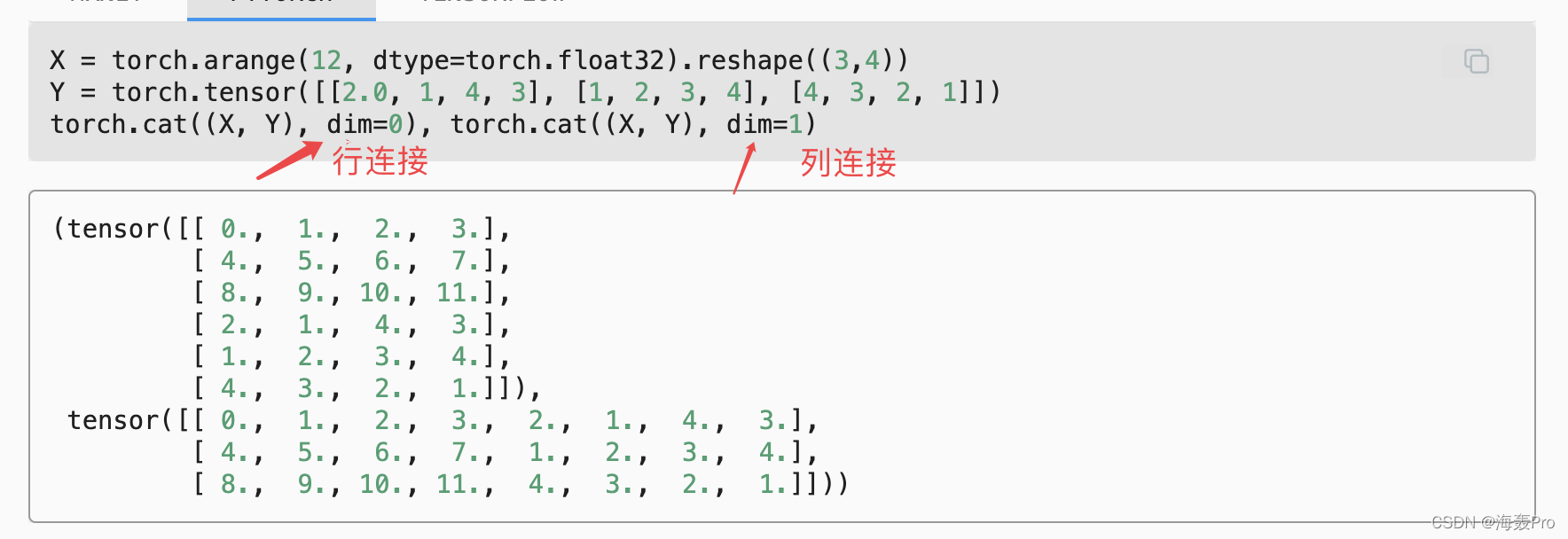### ==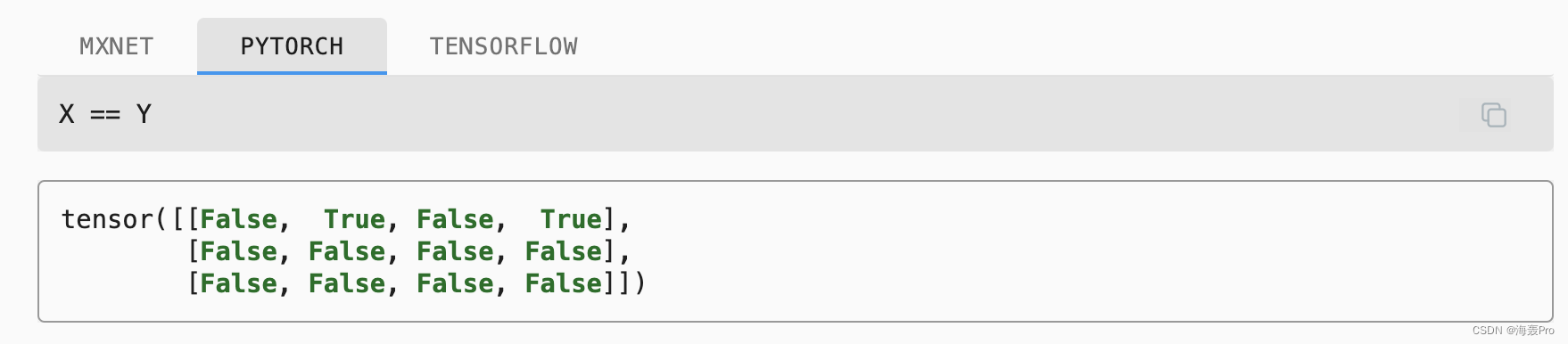### sum()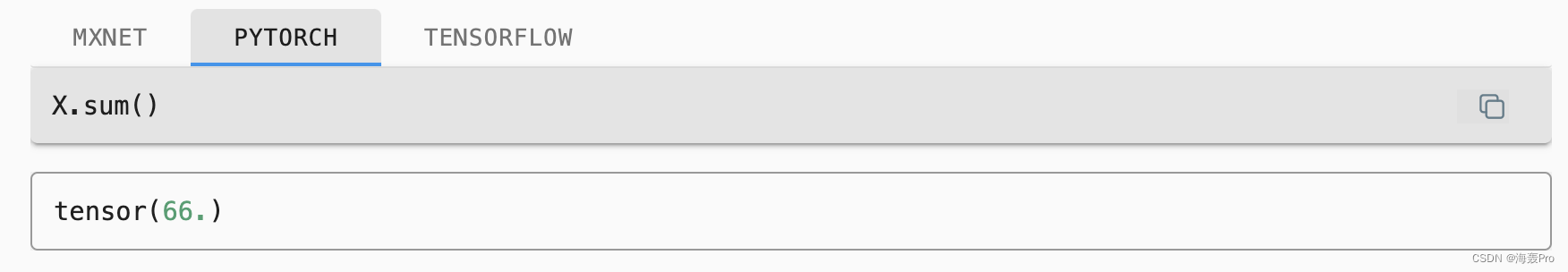## 2.1.3. 廣播機制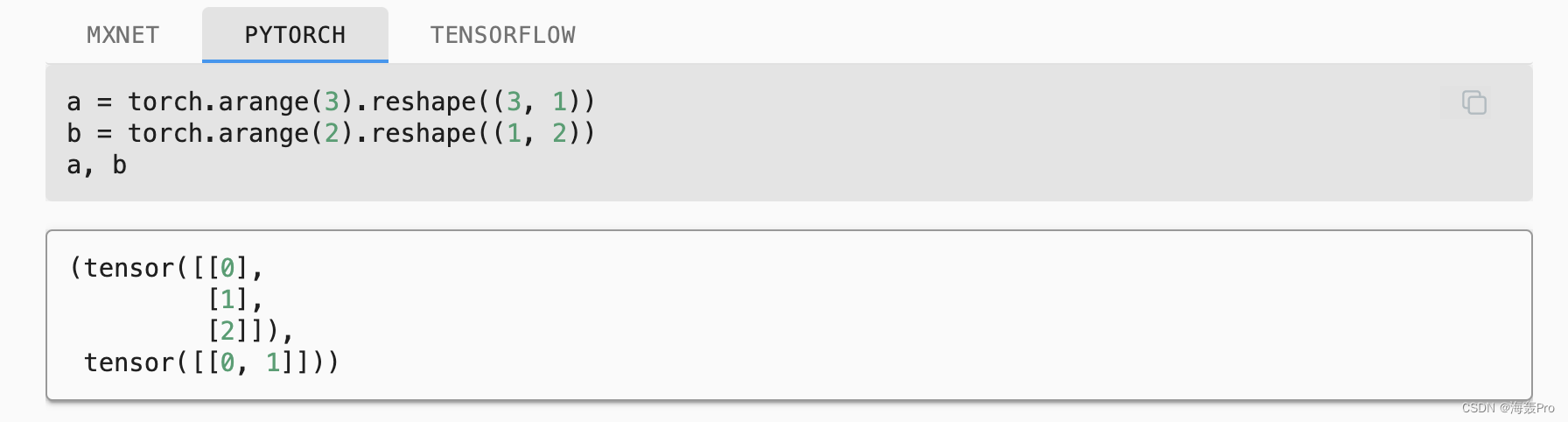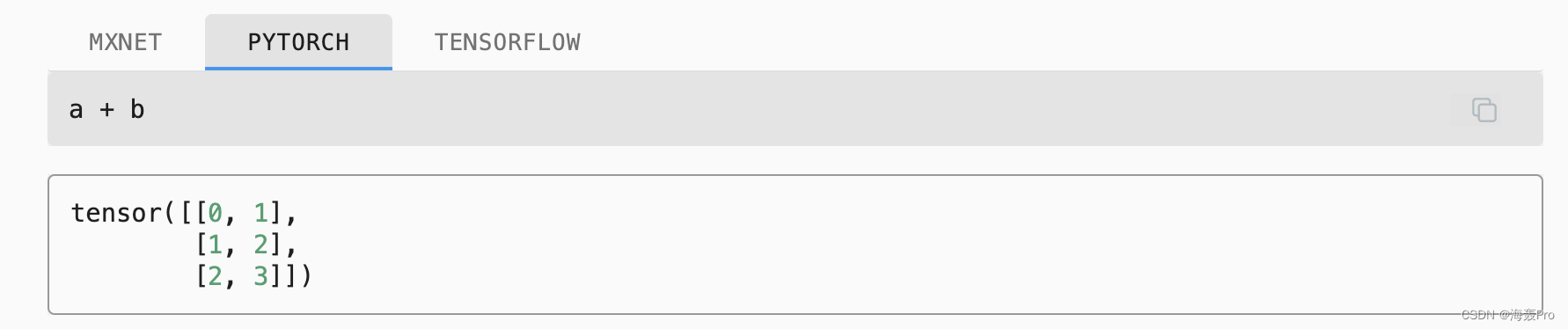```python a是3行1列， b是1行三列 a與b相加時 a變為3行2列（擴列），b也變為3行2列（擴行），再相加（對應位置元素相加）```

## 2.1.4. 索引和切片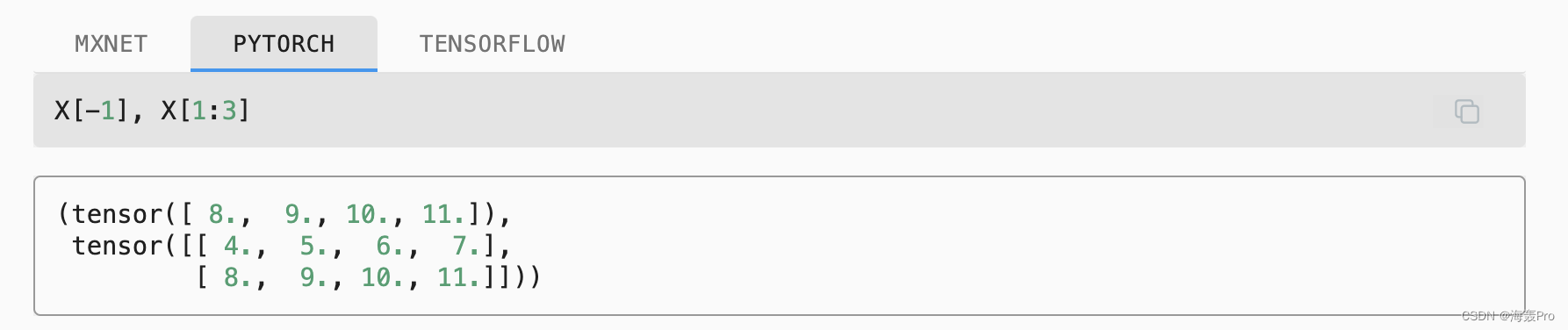X[1:3]：[1,3)，第一行、第二行（不包括第三行）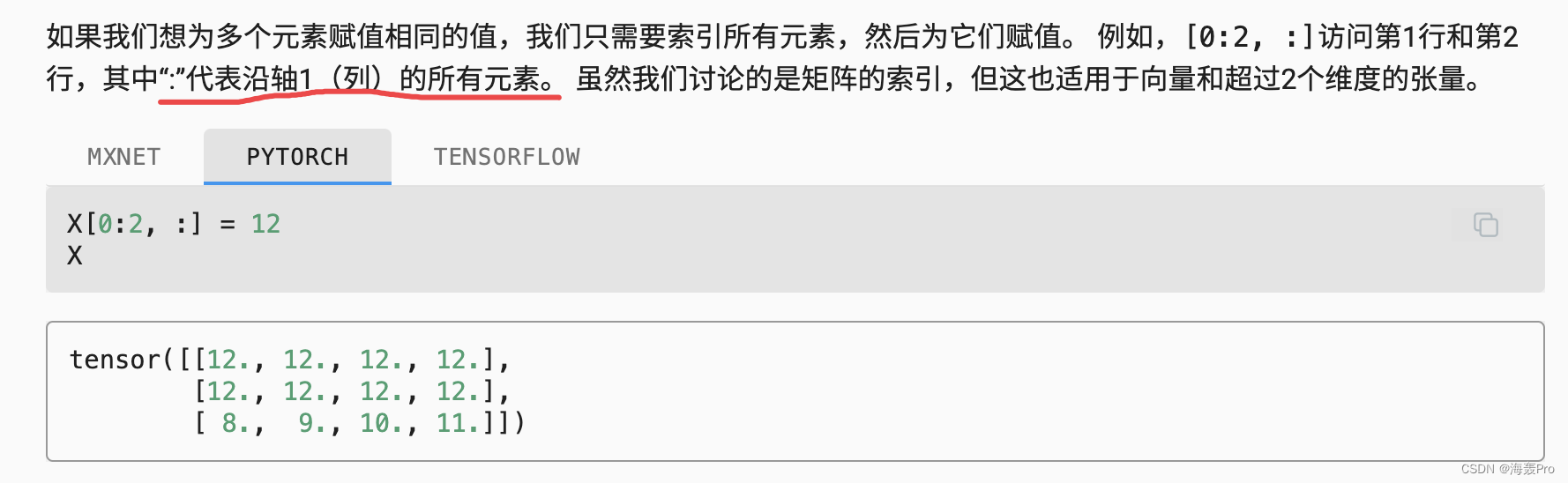## 2.1.5. 節省記憶體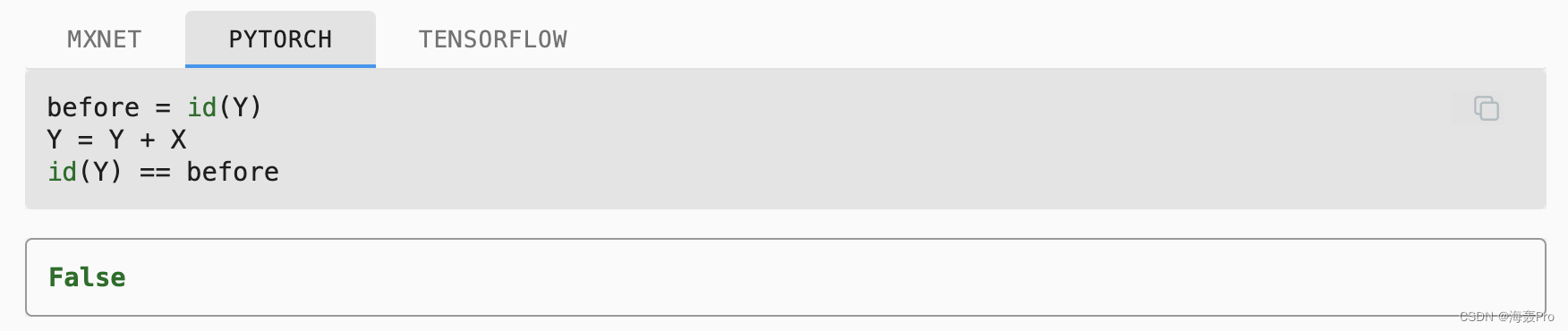id():查詢變數在記憶體中的位置

```python X = torch.arange(3) Y = torch.arange(3) before = id(Y) Y = Y + X id(Y) == before

# False

`使用`Y[:] = Y + X``python X = torch.arange(3) Y = torch.arange(3) before = id(Y) Y[:] = Y + X id(Y) == before

# True

```

```python X = torch.arange(3) Y = torch.arange(3) before = id(Y) Y += X id(Y) == before

# True

```

## 2.1.6. 轉換為其他Python物件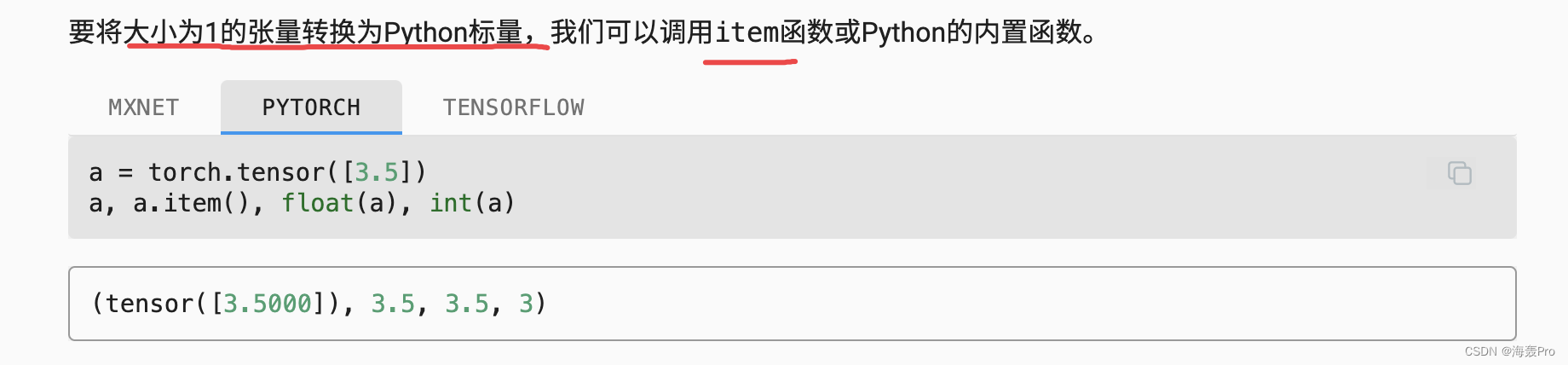# 結語